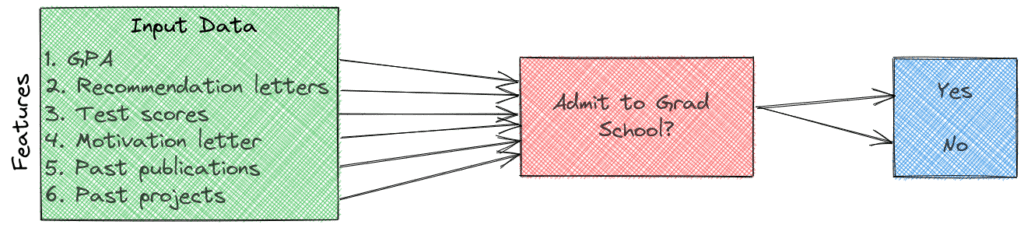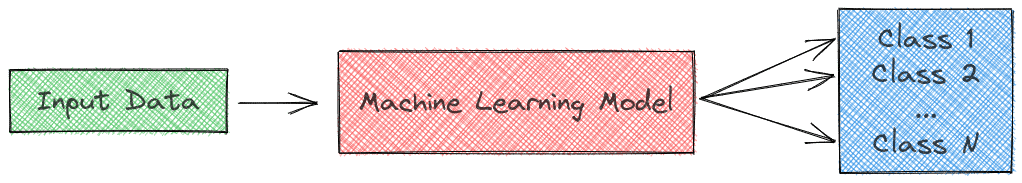## 1. Overview

In this tutorial, we’ll talk about three key components of a Machine Learning (ML) model: Features, Parameters, and Classes.

## 2. Preliminaries

Over the past years, the field of ML has revolutionized many aspects of our life from engineering and finance to medicine and biology. Its applications range from self-driving cars to predicting deadly diseases such as cancer. Generally, the goal of ML is to understand the structure of data and fit that data into models that can be understood and utilized by people. These models are mathematical representations of real-world processes and are divided into:

• supervised where we use labeled datasets to train algorithms into classifying data or predicting outcomes accurately.
• unsupervised where we analyze and cluster unlabeled datasets without the need for human intervention.

## 3. Features

First, let’s talk about features that act as input to the model. Features are individual and independent variables that measure a property or characteristic of the task. Choosing informative, discriminative, and independent features is the first important decision when implementing any model. In classical ML, it is our responsibility to hand-craft and choose some useful features of data while in modern deep learning, the features are learned automatically based on the underlying algorithm.

To better explain the concept of features let’s imagine that we want to implement a model that predicts if a student will be accepted for graduate studies in a university. To choose our features, we should think of variables that are correlated with the outcome meaning that they influence the outcome of a grad application. For example, we can have the following features:

• The GPA of their undergraduate studies
• The recommendation letters of their previous professors or employers
• Their scores in standardized tests like GRE, GMAT, and more
• If they have previous publications
• The projects they worked on during undergradSince the result (accepted or not) depends more or less on all of these variables, they can be used as input features to the ML model. In case we have too many features, we can use feature selection methods.

## 4. Parameters

The next step is to choose the ML model that we will train for our task. Model parameters are defined as the internal variables of this model. They are learned or estimated purely from the data during training as every ML algorithm has mechanisms to optimize these parameters.

Training typically starts with parameters being initialized to some values. As training progresses, the initial values are updated using an optimization algorithm (e.g. gradient descent). The learning algorithm is continuously updating the parameter values as learning progress. At the end of the learning process, model parameters are what constitute the model itself.

To better understand the concept of parameters let’s see what are the parameters in some common ML models:

• In a simple Linear Regression model, wherethe variablesandare the parameters of the model.
• In a Neural Network model, the weights and biases are the parameters of the model.
• In a Clustering model, the centroids of the clusters are the parameters of the model.

We often confuse parameters with hyperparameters. However, hyperparameters are not learned during training by the model but are set beforehand. For example, in clustering, the number of clusters is a hyperparameter while the centroids of the clusters are parameters.

## 5. Classes

Our last term applies only to classification tasks where we want to learn a mapping function from our input features to some discrete output variables. These output variables are referred to as classes (or labels):In our previous task of grad application, we have only two classes that are “Accepted” and not “Not Accepted”.

## 6. Conclusion

In this article, we talked about three key components of ML models: Features, Parameters, and Classes. First, we defined them and then we presented some practical examples.